#### 期刊菜单

Bayesian Network Modeling of Mission Reliability for Clustered UAVs
DOI: 10.12677/CSA.2020.108151, PDF, HTML, XML, 下载: 211  浏览: 517

Abstract: As an important part of modern equipment system, UAV has the characteristics of low cost, dexterous target and low risk. In recent years, the emergence of clustered UAVs has further demonstrated the cost and quantity advantages of UAVs in future wars. However, compared with man-machine, the reliability of cluster UAV has attracted much attention due to the incomplete maturity of UAV technology, more hostile mission environment, complex and diverse relationships among UAV. At the same time, compared with traditional reliability analysis methods such as fault tree, Bayesian network has more advantages in the study of complex systems. In this paper, a reliability modeling method of cluster UAV based on Bayesian network is proposed. This paper proposes the computing logic of the reliability of cluster UAV from three levels of single UAV, UAV subgroup and UAV cluster. According to the modeling process of Bayesian network, the Bayesian network of cluster UAV is es-tablished. The work of this paper provides reference for the subsequent research on the reliability of cluster UAV.

1. 引言

2. 集群无人机

“集群无人机”是多架无人机的聚合过程，在通信网络的支持下，每架无人机共享信息，形成“无人机子群”乃至“无人机集群”，进一步发挥无人机的数量和成本优势。“无人机子群”是多架无人机的集合，无人机通过一定的规则组合起来弥补各自功能上的缺陷，使作战目的得以实现。“无人机子群”是多架无人机协同飞行，共同执行侦察、作战支援、直接打击等作战任务，相较于单无人机能够提高执行单次作战任务的任务成功率。

3. 集群无人机的任务可靠性分析

3.1. 单无人机的任务可靠性分析

${P}_{ri}={k}_{1}\cdot {k}_{2}\cdot {k}_{3}\cdot {P}_{i}$ (1)

3.2. 无人机子群的任务可靠性分析

3.2.1. 层级式子群的任务可靠性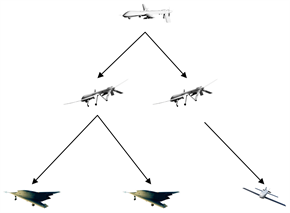Figure 1. UAVs subgroup with hierarchical structure

${P}_{a}={P}_{i1}\cdot {P}_{i2}^{2}\cdot \left(1-{\left(1-{P}_{i3}\right)}^{2}\right)\cdot {P}_{i4}$ (2)

3.2.2. 集中式子群的任务可靠性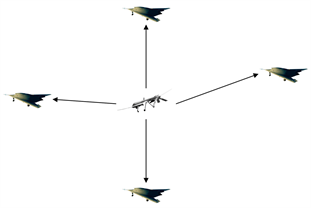Figure 2. UAVs subgroup with centralized structure

${P}_{b}={P}_{i1}\cdot \left(4{P}_{i2}^{3}-3{P}_{i2}^{4}\right)$ (3)

3.2.3. 分布式子群的任务可靠性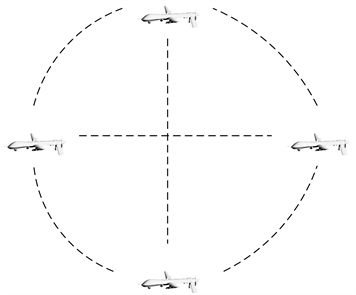Figure 3. UAVs subgroup with distributed structure

${P}_{c}=4{P}_{i}^{3}-3{P}_{i}^{4}$ (4)

3.3. 无人机集群的任务可靠性分析

${P}_{u}={P}_{a}\cdot {P}_{b}\cdot {P}_{c}$ (5)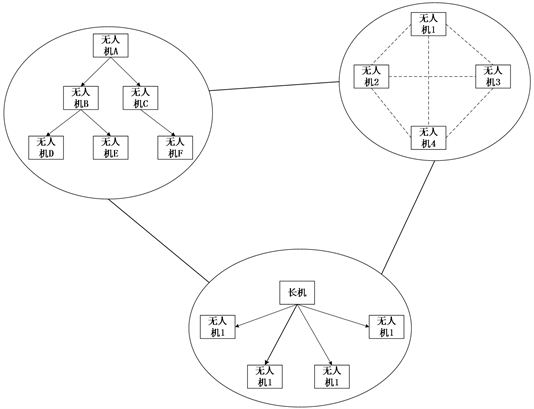Figure 4. Network structure of UAV cluster

4. 贝叶斯网络模型

4.1. 贝叶斯网络概述

${\theta }_{{x}_{i}|{\pi }_{i}}={p}_{B}\left({x}_{i}|{\pi }_{i}\right)$ (6)

$\underset{i=1}{\overset{n}{\prod }}{p}_{B}\left({x}_{i}|{\pi }_{i}\right)=\underset{i=1}{\overset{n}{\prod }}{\theta }_{{x}_{i}|{\pi }_{i}}$ (7)

4.2. 集群无人机任务可靠性的贝叶斯网络建立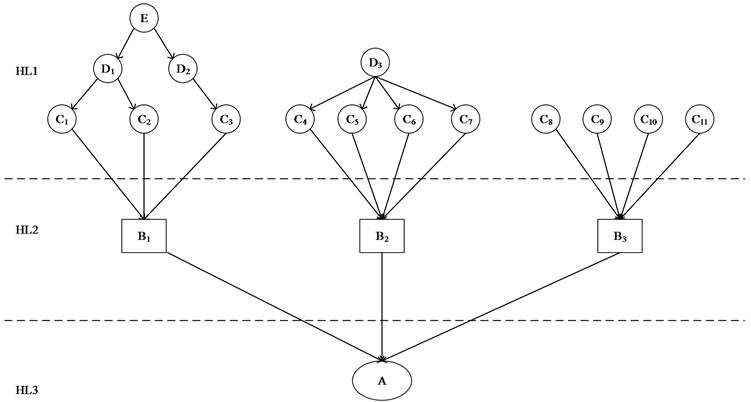Figure 5. Bayesian network model of clustered UAVs

4.3. 集群无人机任务可靠性的贝叶斯网络推理

$p\left({B}_{1}\right)=p\left(E\right)\cdot \underset{i=1}{\overset{2}{\prod }}p\left({D}_{i}|E\right)\cdot \underset{i=1}{\overset{2}{\prod }}p\left({C}_{i}|{D}_{1}\right)\cdot p\left({C}_{3}|{D}_{2}\right)\cdot p\left({B}_{1}|{C}_{1},{C}_{2},{C}_{3}\right)$ (8)

B2代表集中式无人机子群，在这种结构中，根节点D3和子元件C4，C5，C6，C7并联组成多态并联系统。从数学的角度来说，像这种一对多的关系模型，属于最基本的映射关系，因此具有非常广泛的应用，和朴素贝叶斯分类器结构十分相似，假设C4，C5，C6，C7条件独立，集中式无人机子群满足：

$p\left({B}_{2}\right)=p\left({D}_{3}\right)\cdot \underset{i=4}{\overset{7}{\prod }}p\left({C}_{i}|D\right)\cdot p\left({B}_{2}|{C}_{4},{C}_{5},{C}_{6},{C}_{7}\right)$ (9)

$p\left({B}_{3}\right)=\underset{i=8}{\overset{11}{\prod }}p\left({C}_{i}\right)\cdot p\left({B}_{3}|{C}_{8},{C}_{9},{C}_{10},{C}_{11}\right)$ (10)

B1，B2，B3最后构成无人机集群A，其任务可靠性满足：

$p\left(A\right)=\underset{i=1}{\overset{3}{\prod }}p\left({B}_{i}\right)\cdot p\left(A|{B}_{1},{B}_{2},{B}_{3}\right)$ (11)

5. 计算结果分析

(1) 提高单无人机关键部件的可靠性，特别是容易受到电子干扰和恶劣天气影响的部件。单无人机是集群无人机的最小单元，提高单无人机的可靠度，特别是在集群无人机中处于关键地位的无人机，对于提高整个系统的任务可靠度具有促进作用。

(2) 增加无人机子群中并联子无人机的数量。这 样可以提高无人机子群的冗余度，从而提高整个系统的任务可靠度。

(3) 增加并联的无人机子群的数量。增加无人机子群的数量，能大幅度提高任务成功率，从而提高集群无人机的任务可靠性。

6. 结束语

  贾永楠, 田似营, 李擎. 无人机集群研究进展综述[J]. 航空学报, 2019, 40(x): 1-12.  Camille Alain Rabbath, Nicolas Lechevin, 拉巴特, 等. 协同无人机系统安全性与可靠性[M]. 北京: 国防工业出版社, 2015.  全少理, 薛俞, 李秋燕. 基于贝叶斯网络柔性多状态开关可靠性建模与分析[J]. 华北电力大学学报(自然科学版), 2019, 46(5): 25-35.  罗德林, 徐扬, 张金鹏. 无人机集群对抗技术新进展[J]. 科技导报, 2017(7): 28-33.  颜骥, 李相民, 刘立佳, 刘波. 机群多编队作战网络自同步[J]. 系统工程与电子技术, 2014, 36(8): 1566-1572.  王双成. 贝叶斯网络学习、推理与应用[M]. 上海: 立信会计出版社, 2015.